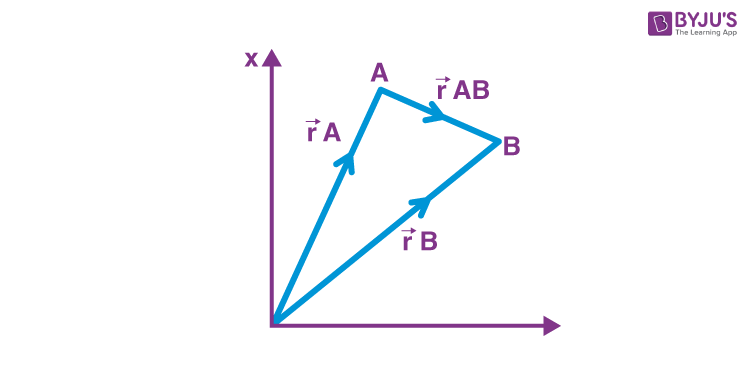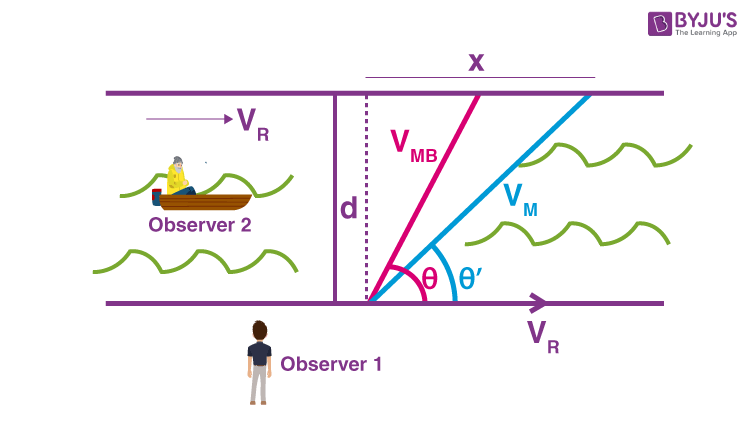# Important Relative Motion Formulas for JEE Main and Advanced

Relative motion is a combined property of the object under study as well as the observer. There is no such thing like absolute motion or absolute rest. Motion is always defined with respect to an observer or reference frame. Having said that, here in this page students will find a list of relative motion formulas which will further boost their revision before the entrance exams.

## Relative Motion in One Dimension

1. Relative Position

$\vec{X}_{AB}=\vec{X}_{A}-\vec{X}_{B}$

Here $\vec{X}_{A}$ and $\vec{X}_{B}$ are the position vector of A and B with respect to the origin

2. Relative Velocity

$\vec{V}_{AB}=\vec{V}_{A}-\vec{V}_{B}$

Here $\vec{V}_{A}$ and $\vec{V}_{B}$ are the velocity w.r.t to ground

$\vec{V}_{AB}$ is the velocity of A w.r.t B. All Velocities are relative and will not have any significance unless the observer is specified. However, when we say “velocity of A” it means the velocity of A w.r.t to the ground which is assumed to be at rest.

3. Relative Acceleration

It is the rate at which relative velocity is changing

$\vec{a}_{AB} = \frac{d}{dt} \vec{V_{AB}} = \frac{d}{dt} \vec{V_{A}} = \frac{d}{dt} \vec{V_{B}}$

4. Equation of motion (if relative acceleration is constant)

v = u+at

S = ut+(1/2)at2

v2 = u2+2as

5. Velocity of Approach/Separation

It is the component of the relative velocity of one particle w.r.t another along the line joining them. If the separation is decreasing, we say it is the velocity of approach and if separation is increasing, then we say it is the velocity of separation.

## Relative Motion in two Dimension$\vec{r}_{AB}= \vec{r}_{A}-\vec{r}_{B}$

here

$\vec{r}_{A}$ , $\vec{r}_{B}$ are position vector of A and B w.r.t origin

$\vec{V}_{AB}=\vec{V}_{A}-\vec{V}_{B}$

Here $\vec{V}_{A}$, $\vec{V}_{B}$ are the velocity w.r.t to ground

$\vec{a}_{AB} = \frac{d}{dt} \vec{V_{AB}} = \frac{d}{dt} \vec{V_{A}} – \frac{d}{dt} \vec{V_{B}}$$\vec{a}_{A}$ – $\vec{a}_{B}$

Here $\vec{a}_{A}$,$\vec{a}_{B}$ are the acceleration w.r.t to ground

### Relative Motion in River Flow

Consider a man swimming in a river with velocity VMR relative to the river at an angle of θ with the river flow. The velocity of the river is VR. Let us consider 2 observers (observer 1 and observer 2). The observer 1 is on the ground and observer 2 is in a raft floating along with the river. Therefore the motion w.r.t to the observer 2 is the same as the motion w.r.t to the river. The man will flow with angle θ with the river flow for observer 2. For observer 1 the velocity of the river will be VM=VMR+VR. Therefore the swimmer will appear to move at an angle θ’ with the river flow.1. Drift

It is defined as the displacement of the man in the direction of the river flow. In the figure above x is the drift.

2. Crossing the river in the shortest time

Time to cross the river will be minimum if man swims perpendicular to the river flow

Tmin = d/VMR

3. Crossing the river in the shortest path, Minimum drift

The minimum possible drift is zero. In this case, the man swims in the direction perpendicular to the river flow as seen from the ground. This path is known as the shortest path. For minimum drift, the man must swim at some angle Φ with the perpendicular in a backward direction, which is given by sin Φ = VR/VMR

Time to cross the river along the shortest path

t = d/VMR cos Φ

$t=\frac{d}{\sqrt{V^{2}_{MR}-V^{2}_{R}}}$

### Wind Airplane Problem

The velocity of the aeroplane with respect to the wind is

$\vec{V}_{AW}=\vec{V}_{A}-\vec{V_{W}}$

Here $\vec{V}_{A}$ is the aeroplane w.r.t the ground, $\vec{V}_{W}$

is the velocity of the wind.

### Rain Problem

The rain is falling vertically with a velocity VR and the observer is moving horizontally with velocity VM, the velocity of rain relative to an observer will be

$\vec{V}_{RM}=\vec{V_{R}}-\vec{V}_{M}$

And direction θ = tan-1(Vm/VR)

## Relative Motion Videos

### Rain Man ProblemTest Your Knowledge On Important Relative Motion Formulas For Jee!